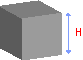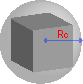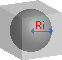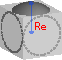# Cube Calculator: Volume, Surface Area, and Sphere Radii

The calculator is designed to provide various measurements related to a cube based on its side length. It can calculate the volume, surface area, circumscribed sphere radius, inscribed sphere radius, and the radius of the sphere tangent to edges.

To use the calculator, simply enter the length of the cube's side, and the calculator will instantly provide the calculated values. This tool is useful for anyone working with cubes, including architects, engineers, and students studying geometry or 3D modeling.

Side length
Digits after the decimal point: 5
Volume

Surface area

Radius of sphere tangent to edges (Re)Volume:
Surface area:Radius of sphere tangent to edges:URL copiado para a área de transferência